# ACU-T: 5301 Ship Hull Dynamics

This tutorial provides the instructions for setting up, solving, and viewing results of a flow around a freely floating ship hull. In this simulation, a wave hits the ship hull and displacement of the ship and the flow around the ship are simulated. This tutorial is designed to introduce you to a number of modeling concepts necessary to perform Free-Surface simulations.

The basic steps in any CFD simulation are shown in ACU-T: 2000 Turbulent Flow in a Mixing Elbow. The following additional capabilities of AcuSolve are introduced in this tutorial:
• Use of Rigid Body type mesh motion
• Use of Free surface and Guide surface capabilities in conjunction of Rigid Body mesh motion.
In this tutorial you will do the following:
• Analyze the problem
• Start AcuConsole and create a simulation database
• Set general problem parameters
• Use the pre-defined material model - Aluminum
• Create Rigid Body Mesh Motion
• Apply Mesh Motion to the Volume and Surface attributes
• Run AcuSolve
• Monitor the solution with AcuProbe
• Post-processing the nodal output with AcuFieldView

## Prerequisites

In order to run this tutorial, you should have already run through the introductory tutorials, ACU-T: 2000 Turbulent Flow in a Mixing Elbow and ACU-T: 5300 Ship Hull Static, and be familiar with AcuConsole, AcuSolve, or AcuFieldView. In order to run this tutorial, you will need access to a licensed version of AcuSolve.

Prior to running through this tutorial, copy AcuConsole_tutorial_inputs.zip from <Altair_installation_directory>\hwcfdsolvers\acusolve\win64\model_files\tutorials\AcuSolve to a local directory. Extract Ship_hull_static.acs and wave_c from AcuConsole_tutorial_inputs.zip.

## Analyze the Problem

The problem to be addressed in this tutorial is shown below. It is a mid-section of a Wigley Ship model. Wigley hulls have been widely used as test cases for evaluating hydrodynamic behavior of ships. The present tutorial demonstrates the simulation of gravity waves hitting a freely floating Wigley hull and evaluating the position of the hull with time. This tutorial is similar to the Ship Hull Static tutorial, except that the ship hull is freely floating as a rigid body compared to a static ship hull.

Since the motion considered in this tutorial is perpendicular to the length of the ship, an analysis of a 2D section of the ship hull would be appropriate with lesser computation time without compromising on accuracy. The mid-section dimensions of the Wigley hull is a function of total ship length and the model used in this tutorial is the mid-section of Wigley hull whose ship length is 1m.

### Generation of Surface Gravity Waves

Wind-generated gravity waves on the free surface of the sea are generated using a UDF (User-Defined Function).

A linear solution of surface gravity wave propagation would result in the following equation for horizontal velocity of wave.

$u={U}_{0}+U\frac{\mathrm{cosh}k\left(D-z\right)}{\mathrm{sinh}\left(kD\right)}\text{cos}\left(\omega t-kx\right)$

Where,

$u$ is the horizontal particle velocity of wave

${U}_{0}$ is the speed of the wave

U is the velocity amplitude of disturbance

$k$ is wave number = $2\pi /\lambda$

$\lambda$ is the wave length of the wave

$D$ is the depth of the water

$\omega$ is the frequency of the wave = $\frac{2\pi }{T}$

$T$ is the time period of the wave

t is the time

In the present simulation, the following values are used for the variables of above equation:

U = 0.1256 m/s

$T$ = 1.0 sec

$k$ = 12.566 m-1

${U}_{0}$ = 0.01 m/s

$D$ = 0.5 m

In the present tutorial, at the inlet you will generate the wave for 2 seconds and simulate the motion of the wave for 5 seconds. A UDF (wave.c) written in C language is used for this purpose. For the details of the functions used in the wave.c, refer to the AcuSolve User-Defined Functions Manual.

### Two Dimensional Simulations in AcuSolve

AcuSolve does not support a CFD analysis on a 2D surface mesh. However, a 2D analysis can be simulated on a volume mesh by having a single element extrusion along the perpendicular direction of 2D surface of interest and then having identical boundary conditions of symmetry or slip on both sides of extrusion. This way you can ensure that the solution does not vary along the thickness (extrusion), which is essentially a 2D representation of the problem. The present tutorial uses this approach.

### Modeling the Solid Ship Hull

For the present tutorial the following properties are assumed for the ship hull.

Material = Aluminum

Density = 2702 kg/m3

Body Center = (1.12971, 0.0, 0.0082984) m

Body Mass = 0.2038 kg

Body Weight = 0.2038 × 9.81 = 2.0 N

Components of Moments of Inertia (in kg m2):

${I}_{xx}$ = 0.00113969

${I}_{yy}$ = 0.0051392

${I}_{zz}$ = 0.004006

${I}_{xy}={I}_{yx}=0$

${I}_{yz}={I}_{zy}=0$

${I}_{zx}={I}_{xz}=0$

### Rigid Body Dynamic Analysis

The rigid body is driven by the sum of two kinds of forces and moments.
• Internal Forces/Moments: refers to the integrated fluid traction and moment
• External Forces/Moments: refers to the force and moment you specify

A local coordinate system is used to simplify the definition of the rigid body model and the solution of the equations of motion. The translational and rotational equations of motion are:

Where,

are the translational displacement, velocity and acceleration vectors, respectively as:

$x=\left[\begin{array}{c}x\\ y\\ z\end{array}\right]$, $v=\left[\begin{array}{c}{v}_{x}\\ {v}_{y}\\ {v}_{z}\end{array}\right]$, $a=\left[\begin{array}{c}{a}_{x}\\ {a}_{y}\\ {a}_{z}\end{array}\right]$

are the angular displacement, angular velocity and angular acceleration vectors, respectively as:

$\theta =\left[\begin{array}{c}{\theta }_{x}\\ {\theta }_{y}\\ {\theta }_{z}\end{array}\right]$,$\omega =\left[\begin{array}{c}{\omega }_{x}\\ {\omega }_{y}\\ {\omega }_{z}\end{array}\right]$, $\alpha =\left[\begin{array}{c}{\alpha }_{x}\\ {\alpha }_{y}\\ {\alpha }_{z}\end{array}\right]$

$m$ is the mass of the body.

$I=\left[\begin{array}{ccc}{I}_{xx}& {I}_{xy}& {I}_{xz}\\ {I}_{yx}& {I}_{yy}& {I}_{yz}\\ {I}_{zx}& {I}_{zy}& {I}_{zz}\end{array}\right]$ is the dyadic (moment of inertia) matrix of the body.

are the translational and rotational damping matrices, respectively.

are the translational and rotational stiffness matrices, respectively.

are the internal Forces and Moments from the fluid.

are the external Forces and Moments you specify.

### Rigid Body Dynamics Analysis for a 2D Problem

1. The displacements of the ship along its span (y-direction) is neglected.
2. The axis of rotation for the ship is along the y-direction and the variables along other axis are neglected.
3. Translational damping and stiffness matrices and their rotational equivalents are assumed to be zero.
4. The only external force acting on the rigid body is the gravity force (${F}_{g}$) along the z-axis.
5. The external moments acting on the body is zero, because the only external force acting on the body is gravity which does not generate any moment.

For the present tutorial, a single element extrusion will be made along the y-axis. Based on the above assumptions, you arrive with:

$x=\left[\begin{array}{c}x\\ 0\\ z\end{array}\right]$, $v=\left[\begin{array}{c}{v}_{x}\\ 0\\ {v}_{z}\end{array}\right]$, $a=\left[\begin{array}{c}{a}_{x}\\ 0\\ {a}_{z}\end{array}\right]$

$\theta =\left[\begin{array}{c}0\\ {\theta }_{y}\\ 0\end{array}\right]$, $\omega =\left[\begin{array}{c}0\\ {\omega }_{y}\\ 0\end{array}\right]$, $\alpha =\left[\begin{array}{c}0\\ {\alpha }_{y}\\ 0\end{array}\right]$

The translational equations of the motion will be:

$m\left[\begin{array}{c}{a}_{x}\\ 0\\ {a}_{z}\end{array}\right]=\left[\begin{array}{c}{F}_{I}{}_{x}\\ {F}_{I}{}_{y}\\ {F}_{I}{}_{z}+{F}_{g}\end{array}\right]$

The rotational equations of the motion will be:

The only critical component of moment of inertia for the present tutorial is ${I}_{yy}$.

## Define the Simulation Parameters

### Start AcuConsole and Open the Simulation Database

In the next steps you will start AcuConsole and open a database that is set up for a Ship hull static simulation. You will then make appropriate changes to the database to take into account the dynamics of the ship motion.

1. Start AcuConsole from the Windows Start menu by clicking Start > Altair <version> > AcuConsole.
2. Click the File menu, then click Open to open the Choose a file dialog.
Note: You can also open the Choose a file dialog by clicking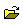on the toolbar.
3. Browse to the directory where you saved Ship_hull_static.acs.
This will be your working directory.
4. Click Ship_hull_static.acs to open the database.
5. Click File > Save As and enter Ship_hull_dynamics as the file name for the database.
6. Click Save to create the database.

### Set General Simulation Parameters

In the next steps you will modify global attributes needed for the transient portion of the simulation.

1. Click BAS in the Data Tree Manager to switch to basic view in the Data Tree.
2. Double-click the Global Data Tree item to expand it.
Tip: You can also expand a tree item by clicking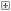next to the item name.
3. Double-click Problem Description to open the Problem Description detail panel.
Tip: You can also open a panel by right-clicking a tree item and clicking Open on the context menu.
4. Name the Sub title as Ship hull dynamics.

### Set Material Model Parameters

AcuConsole has three pre-defined materials, Air, Aluminum, and Water.

In the next steps you will verify that the pre-defined material properties of water and aluminum match the desired properties for this problem.

1. Double-click Material Model in the Data Tree to expand it.
2. Double-click Water in the Data Tree to open the Water detail panel.
The Material type for water is Fluid.
Note: The detail panel can be resized by dragging the right frame of the panel.
3. Click the Density tab. Verify that the density of water is 1000.0 kg/m3.
4. Click the Viscosity tab. Verify that the viscosity of water is 0.001 kg/m-sec.
5. Double-click Aluminum in the Data Tree to open the Aluminum detail panel.

The Material type for aluminum is Solid.

6. Click the Density tab. Verify that the density of aluminum is 2702.0 kg/m3.
7. Save the database to create a backup of your settings. This can be achieved with any of the following methods.
• Click the File menu, then click Save.
• Click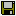on the toolbar.
• Click Ctrl+S.
Note: Changes made in AcuConsole are saved into the database file (.acs) as they are made. A save operation copies the database to a backup file, which can be used to reload the database from that saved state in the event that you do not want to commit future changes.

### Set Mesh Motion Parameters

In the next steps you will define the mesh motion based on the rigid body dynamics of the ship hull.

1. Click ALE in the Data Tree Manager to see all the settings related to mesh motion.
2. Right-click Mesh Motion and click New to create a new mesh motion.
3. Rename Mesh Motion 1 to Rigid_body_mesh_motion.
4. Double-click Rigid_body_mesh_motion and change the Type to Rigid Body Dynamic.
Note: The values assigned to the parameters in this section are the material and geometric properties of the ship hull. Please refer to ACU-T: 5300 Ship Hull Static.
5. Set X displacement and Z displacement as Active.
6. Set Y displacement as Inactive.
7. Set X rotation and Z rotation as Inactive.
8. Set Y rotation as Active.
9. For Mass, enter 0.2038 Kg.
10. Click Open Array adjacent to Center.
11. Enter the coordinates for the center of the ship hull, as shown below, and click OK.
Local Coordinates: This parameter defines the direction of local xyz coordinate system, specified with respect to the global xyz coordinate system.
12. Click Open Array adjacent to Local coordinates.
Since the local and global coordinates are same in this simulation, use the following defaults.
13. Click OK.
14. Click Open Array adjacent to Dyadic, and enter the Moment of Inertia matrix, as shown below.
Though for the present 2D problem the only critical component of moment of inertia is Iyy as mentioned in the section Rigid Body Dynamics Analysis, AcuSolve would require the dyadic matrix to be positive definite because of the 3D volume mesh (refer to 2-Dimensional simulations in AcuSolve) and therefore, requires the input of Ixx, Izz.
The parameters Stiffness, Damping, Rotational stiffness, and Rotational damping will be considered zero, because the ship is assumed to be freely floating on water.
External forces: The force of gravity is the external force acting on the ship hull along the positive-z direction.
15. Click Open Array adjacent to External forces.
16. Enter the Force vector as shown below and click OK.
External Moment: The only external force on the ship hull is force of gravity. It does not produce any moment. Therefore, the External Moment will be zero in this simulation.
The parameters Initial displacement, Initial velocity, Initial rotation, Initial angular velocity, Initial fluid forces, and Initial fluid moments will be considered zero, because of the stationary, equilibrium position of the ship considered at the start of the simulation.
Surface outputs: This parameter lists the array of surfaces whose output of forces and moments will be enforced on the Rigid body (in this case, Ship hull). In this simulation, the forces from the fluid will be enforced on the ship hull through the surface “Hull_guide” of Fluid volume.
17. Click Open Refs adjacent to Surface outputs.
18. In the Reference Editor, click Add Row.
19. Select Hull_guide and click OK.

### Apply Volume Parameters

1. Click BAS in the Data Tree Manager to switch to basic view in the Data Tree.
2. Expand the Model Data Tree item.
3. Expand Volumes.
4. Under Guide_Vol_Ship, double-click Element Set.
5. Change Medium to Solid.
6. Set Material Model to Aluminum.
7. Set Body force to Gravity.
8. Change the Mesh motion to Rigid_body_mesh_motion.

### Set the Mesh Motion to the Guide Surface

1. Click ALE in the Data Tree Manager to see all the settings related to mesh motion.
2. Expand the Model > Surfaces > Guide_surf tree item.
3. Double-click Guide Surface.
4. Change the Mesh motion to Rigid_body_mesh_motion.

### Compile UDF for Windows

A UDF written in C language (wave.c) is provided with the tutorial. Now this C program should be compiled using the following steps.

1. Start AcuSolve Command Prompt from the Windows Start menu by clicking Start > Altair <version> > AcuSolve Cmd Prompt .
2. Change the directory to present working directory using the ‘cd’ command.
The working directory is where all files related to the simulation will be stored. When you are setting up the problem, there will be a file with extension .acs in this directory, which corresponds to an AcuConsole database. Once the mesh and solution are generated, additional files and directories will be added by AcuSolve.
3. Enter the command acuMakeDll –src wave.c.
A set of files necessary for the use of UDF are created.

### Compile UDF for Linux

A UDF written in C language (wave.c) is provided with the tutorial. Now this C program should be compiled using the following steps.

1. In the terminal, use the cd command to change the directory to the current working directory.
2. Enter the command acuMakeLib -src gravity.c.
A set of files necessary for the use of UDF are created.

## Compute the Solution and Review the Results

### Run AcuSolve

In the next steps you will launch AcuSolve to compute the solution for this case.

1. Click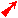on the toolbar to open the Launch AcuSolve dialog.

For this case, the default values will be used.

AcuSolve will run using four processors (if available, higher number of processors may be specified) and AcuConsole will generate AcuSolve input files and will launch AcuSolve. AcuSolve will calculate the transient solution for this problem.

2. Click Ok to start the solution process.

While computing the solution, an AcuTail window opens. Solution progress is reported in this window. A summary of the solution process indicates that the run has been completed.

The information provided in the summary is based on the number of processors used by AcuSolve. If you use a different number of processors than indicated in this tutorial, the summary for your run may be slightly different than the summary shown.

3. Close the AcuTail window and save the database to create a backup of your settings.

### Post-Process with AcuProbe

AcuProbe can be used to monitor various variables over solution time. In the present simulation it is worthwhile to monitor the forces on the ship hull.

1. Open AcuProbe by clicking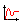on the toolbar.
2. In the Data Tree on the left, expand Surface Output > Hull_guide > Forces and Moments.
3. Right-click on x_wall_shear_stress and click Plot.
Note: You might need to click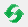on the toolbar in order to properly display the plot.
4. Repeat the above steps for z_wall_shear_stress and click Plot.
5. You can also save the plots as an image.
1. From the AcuProbe dialog, click File > Save.
2. Enter a name for the image and click Save.
6. The time series data of the variables can also be exported as a text file for further post-processing.
1. Right-click on the variable that you want to export and click Export.
2. Enter a File name and choose .txt for the Save as type.
3. Click Save.

### View Results with AcuFieldView

Now that a solution has been calculated, you are ready to view the flow field using AcuFieldView. AcuFieldView is a third-party post-processing tool that is tightly integrated to AcuSolve. AcuFieldView can be started directly from AcuConsole, or it can be started from the Start menu, or from a command line. In this tutorial you will start AcuFieldView from AcuConsole after the solution is calculated by AcuSolve.

In the following steps you will start AcuFieldView, create an animation of the ship hull motion with the contours of z-mesh displacement.

#### Start AcuFieldView

1. Click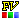on the AcuConsole toolbar to open the Launch AcuFieldView dialog.
2. Click Ok to start AcuFieldView.
When you start AcuFieldView from AcuConsole, the results from the last time step of the solution that were written to disk will be loaded for post-processing.

#### Animate to View Displacement of the Ship

These steps are provided with the assumption that you are able to manipulate the view in AcuFieldView to have a white background, perspective turned off, outlines turned off, and the viewing direction set to +z. If you are unfamiliar with basic AcuFieldView operations, refer to Manipulate the Model View in AcuFieldView.

1. Orient the geometry so you can see the entire model clearly, as shown in the figure below.
2. In the Boundary Surfaces dialog, uncheck Show Mesh.
3. Select z-mesh-displacement as the scalar function and click Calculate.
4. In the Legend tab, click Show Legend.
5. Change the color to black.
Note: You can move the legend using Shift + left-click.
6. From the Colormap tab, turn on Local.
7. Click Tools > Flipbook Build Mode and click OK .
8. Click Tools > Transient Data and move the slider all the way back to reflect the 1st time step data on the boundary surfaces.
9. Click Build to build the animation.
10. Once the animation is built, click on Frame Rate and change it to 0.1.
11. Save the animation as Z-Displacement.mpg.

## Summary

In this tutorial, you worked through a basic workflow to set up a dynamic ship-hull simulation with surface gravity waves. You started with an .acs file from the Ship Hull Static tutorial and modified the set up to accommodate the rigid body motion of the ship hull. Once the case was set up, you generated a solution using AcuSolve. Results were post-processed in AcuFieldView to allow you to create animation of the Ship hull movement with time. New features introduced in this tutorial include: Rigid Body type mesh motion, use of Free surface and Guide surface capabilities in conjunction of Rigid Body mesh motion.